# Arithmetic - math word problems

1. Im>0?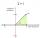Is -10i a positive number?
2. DigitsWrite the smallest and largest 1-digit number.
3. TrioTrio of workers earn 750 euros. Money divided by the work that each of them made​​. First received twice as the second, the second received three times more than the third. How many got everyone from workers?
4. ReciprocalCalculate reciprocal of z=0.8-1.8i:
5. Rotary coneRotary cone whose height is equal to the circumference of the base, has a volume 229 cm3. Calculate the radius of the base circle and height of the cone.
6. Efficiency of rail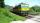With subsidies are business easy. Calculate how much must cost rail ticket (x) in today's ticket price € 11 and if the trains was not subsidized, if it is known that without subsidies would cost three times today's ticket prices. Calculate the value of
7. ClockHow many times a day hands on a clock overlap?
8. R TrapeziumRectangular trapezium has bases 12 and 5 and area 84 cm2. What is its perimeter?
9. InterestCalculate how much you earn for 10 years 43000 deposit if the interest rate is 1.3% and the interest period is a quarter.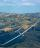Calculate how many g's (gravity accelerations) feel glider pilot when turning the horizontal circles of radius 148 m flying at 95 km/h. Centripetal acceleration is proportional to the square of the speed and inversely proportional to the radius of rotatio
11. Vans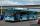In how many ways can 9 shuttle vans line up at the airport?
12. AVG of INT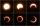What is the average of the integers from 9 throuht 52 inclusive?
13. CenterCalculate the coordinates of the circle center: ?
14. Hours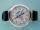How many hours is 9 days?
15. ABS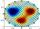What is the value of ? ?
16. Exp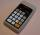If ?, then n is:
17. Candy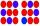How many ways can divide 10 identical candies to 5 children?
18. Reciprocal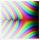What is the reciprocal of the sum of the reciprocals of 4 and 9?
19. Product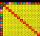The sum and the product of three integers is 6. Write largest of them.
20. Gain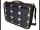Find the gain whenever the output power is 21x the input power.

Do you have an interesting mathematical word problem that you can't solve it? Submit math problem, and we can try to solve it.

We will send a solution to your e-mail address. Solved examples are also published here. Please enter the e-mail correctly and check whether you don't have a full mailbox.

Please do not submit problems from current active competitions such as Mathematical Olympiad, correspondence seminars etc...